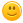#I need to use the number pi in C. How to enter it?

closed Nov 25, 2021
for example y=pi*4?

answered Nov 19, 2021 by (79,800 points)

Well, you know the value of PI, all you have to do is create either a variable or a macro for it.

```#include <stdio.h>

#define PI 3.14159265359f
// float PI = 3.14159265359f; // This would work just as fine

int main()
{
float y = PI * 4.0f;
printf("y = %f\n", y);
return 0;
}```
commented Nov 19, 2021 by (100 points)
i agree with you since you declared the value of PI as a global var it can be used anywhere in the prorgram
+1 vote
answered Nov 24, 2021 by (160 points)

You can also use it directly by adding another library in your code.

The library is math.h, I will demonstrate it by calculating area of a circle, so it would look like,

#include<stdio.h>

#include<math.h>

int main()

{

float r;

printf("Enter the value of radius of circle\n");

scanf("%f",&r);

printf("The area of the circle is %f\n",M_PI*r*r);

return 0;

}

Hope it helps